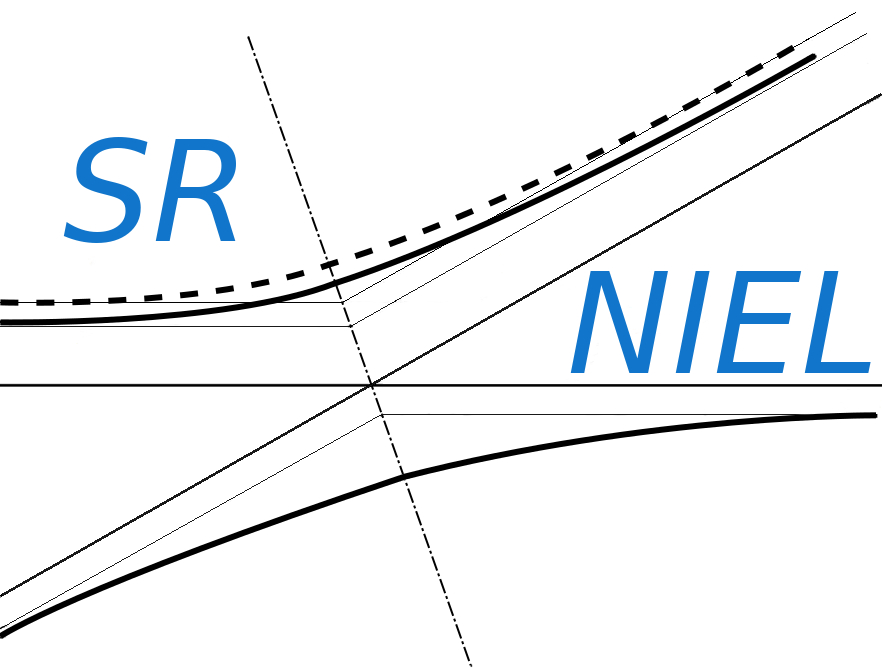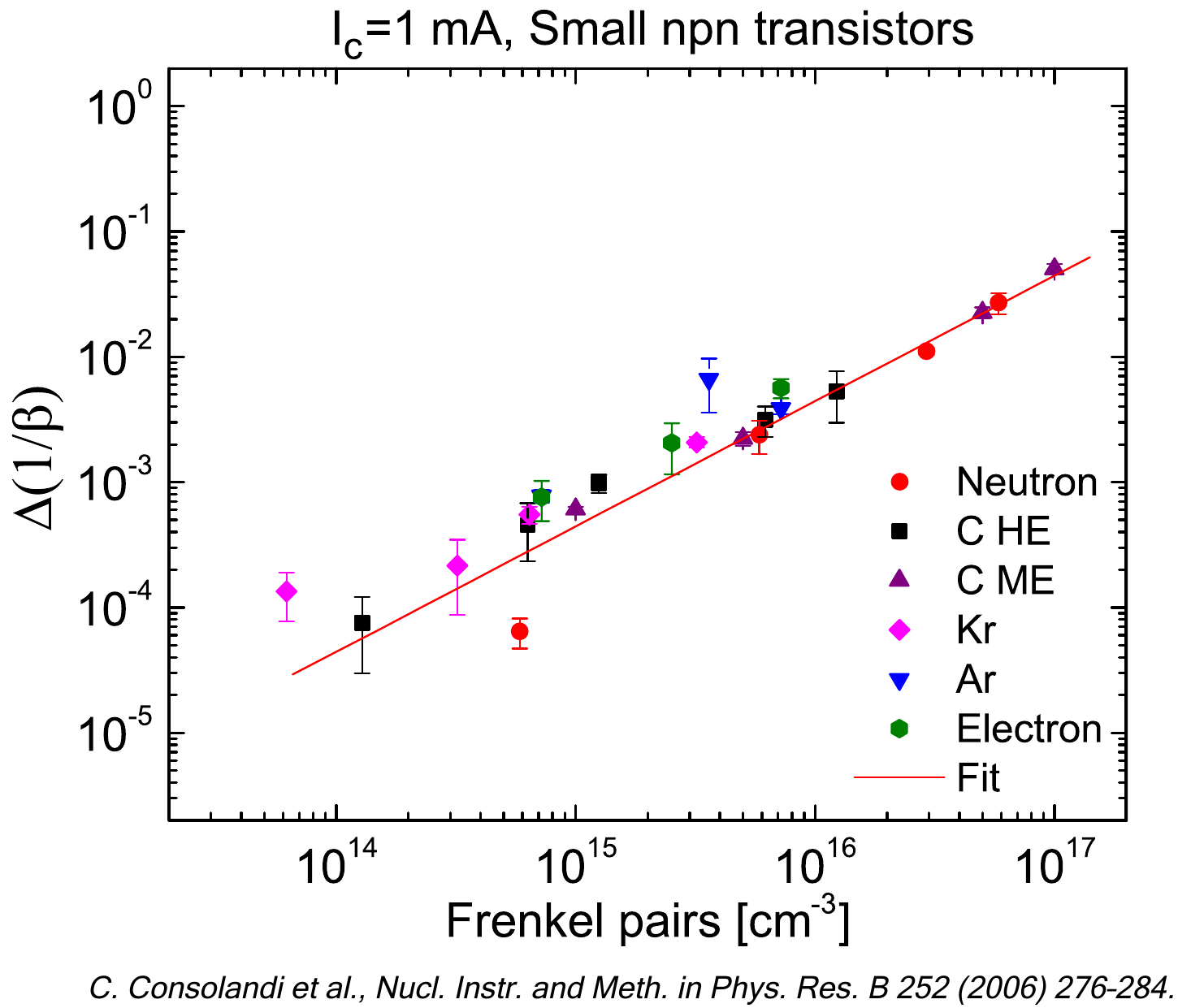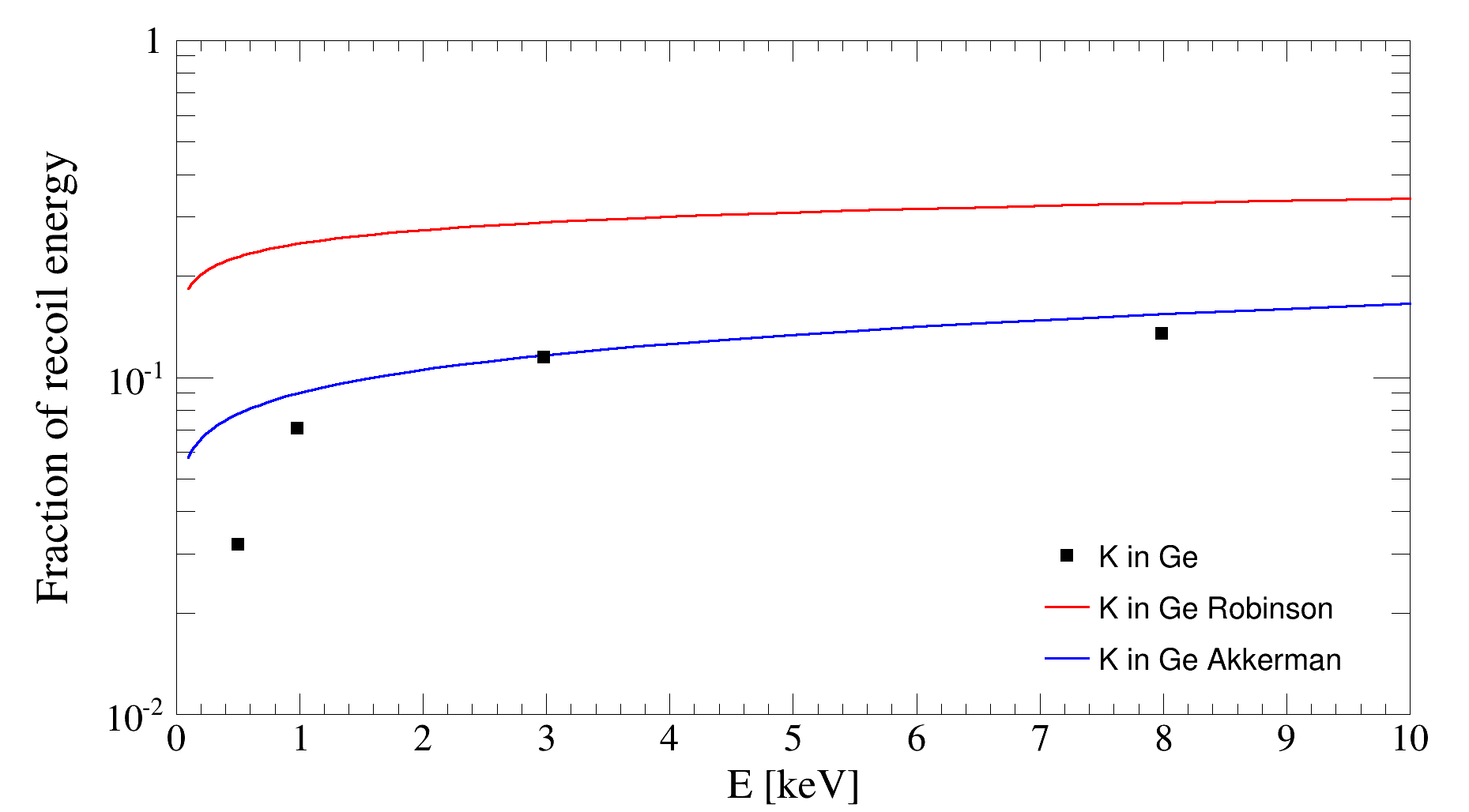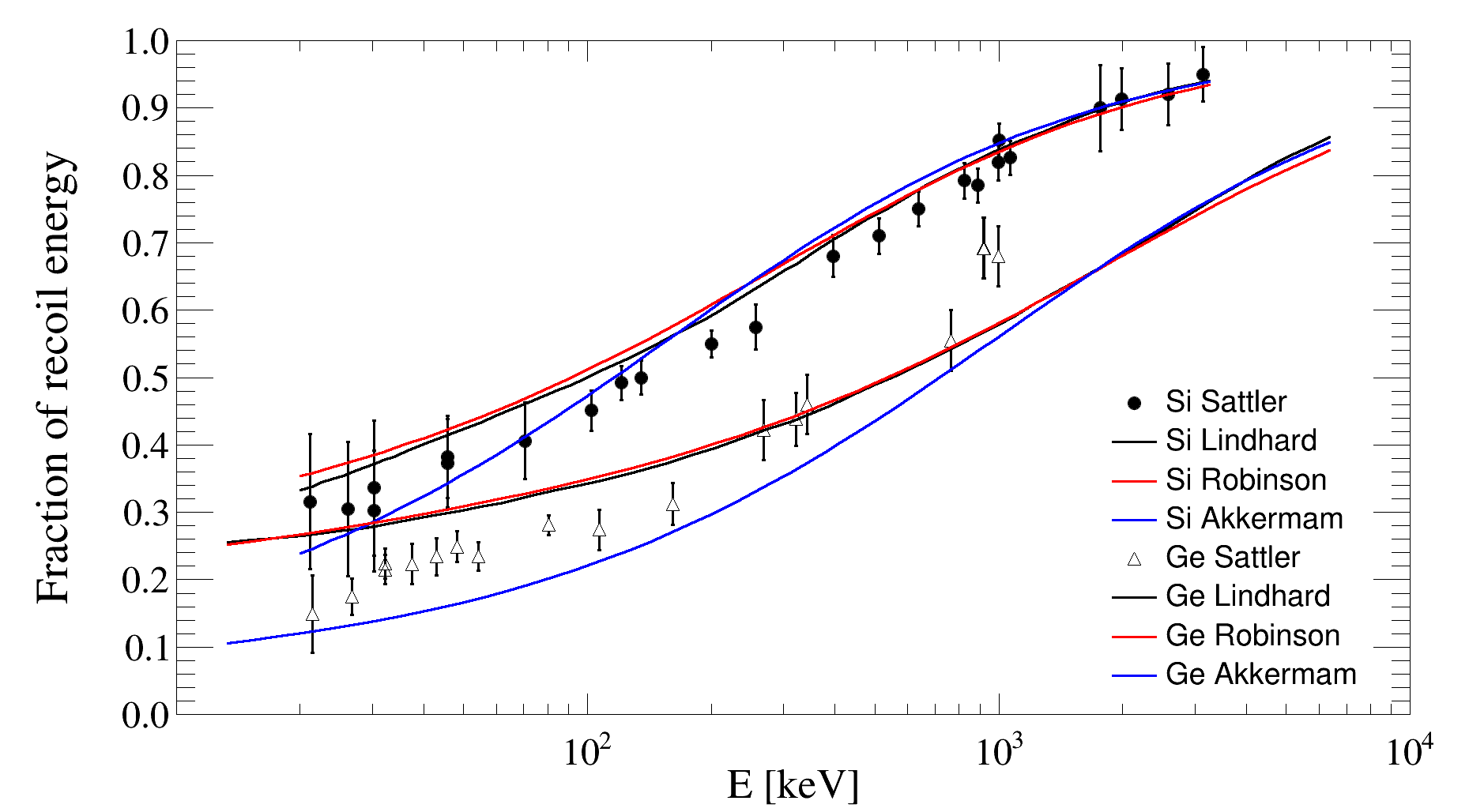SR-NIEL – 7 Screened Relativistic (SR) Treatment for NIEL Dose Nuclear and Electronic Stopping Power Calculator (version 7.5)As discussed in the Energy Partition Function webpage, the partition function gives the fraction of recoil energy that goes into further displacement and can be approximated with Robinson and Akkerman analytical treatment. Here is presented the comparison of the two models with respect experimental data.

In the following graph, experimental points of Ne, O, C recoils in Si can be found in Akkerman and Barak (2006) and are taken from H. O. Funsten et al. (2001) and H. O. Funsten et al. (2004). Data are superimposed to the Akkerman and Robison analytical approximation of the partition function.K recoil in Ge data are found in Akkerman and Barak (2006) and come from I. A. Abroyan et al. (1962)Data points for Si recoils in Si, along with the Lindhard curve, are taken from Sattler (1965). Data and Lindhard curve for Ge recoils in Ge come from Sattler (1966). Robinson and Akkerman analytical approximation are also superimposed:### References

A. Akkerman and J. Barak (2006), IEEE Trans. on Nucl. Sci. vol. 53, 3667.

H. O. Funsten, S. M. Ritzau, R. W. Harper, and R. Korde, IEEE Trans. Nucl. Sci., vol. 48, pp. 1785–1789, 2001.

H. O. Funsten, S. M. Ritzau, and R. W. Harper, Appl. Phys. Lett., vol. 84, pp. 3552–3554, 2004.

I. A. Abroyan and V. A. Zborovskii, Dokl. Akad. Nauk, SSSR, vol. 144, pp. 531–534, 1962; Sov. Phys. Dokl., vol. 7, p. 417, 1962.